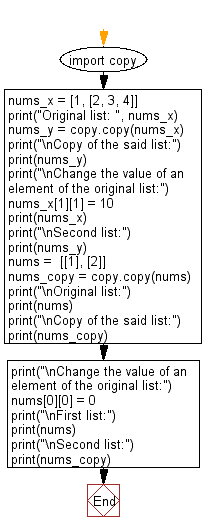﻿ Python: Create a shallow copy of a given list - w3resource# Python: Create a shallow copy of a given list

## Python module: Exercise-1 with Solution

Write a Python program to create a shallow copy of a given list. Use copy.copy

Sample Solution:

Python Code:

``````import copy
nums_x = [1, [2, 3, 4]]
print("Original list: ", nums_x)
nums_y = copy.copy(nums_x)
print("\nCopy of the said list:")
print(nums_y)
print("\nChange the value of an element of the original list:")
nums_x = 10
print(nums_x)
print("\nSecond list:")
print(nums_y)
nums =  [, ]
nums_copy = copy.copy(nums)
print("\nOriginal list:")
print(nums)
print("\nCopy of the said list:")
print(nums_copy)
print("\nChange the value of an element of the original list:")
nums = 0
print("\nFirst list:")
print(nums)
print("\nSecond list:")
print(nums_copy)
```
```

Sample Output:

```Original list:  [1, [2, 3, 4]]

Copy of the said list:
[1, [2, 3, 4]]

Change the value of an element of the original list:
[1, [2, 10, 4]]

Second list:
[1, [2, 10, 4]]

Original list:
[, ]

Copy of the said list:
[, ]

Change the value of an element of the original list:

First list:
[, ]

Second list:
[, ]
```

Flowchart:## Visualize Python code execution:

The following tool visualize what the computer is doing step-by-step as it executes the said program:

Python Code Editor:

Have another way to solve this solution? Contribute your code (and comments) through Disqus.

What is the difficulty level of this exercise?

Test your Python skills with w3resource's quiz

﻿

## Python: Tips of the Day

For-else construct useful when searched for something and find it:

```# For example assume that I need to search through a list and process each item until a flag item is found and
# then stop processing. If the flag item is missing then an exception needs to be raised.

for i in mylist:
if i == theflag:
break
process(i)
else:
raise ValueError("List argument missing terminal flag.")
```